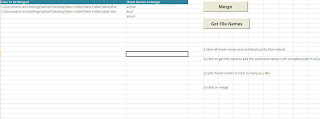## Saturday, July 30, 2011

### Merge Data from multiple workbooks for specific sheets only

If you want to merge data from specific sheets from multiple workbooks into single workbook. For example i have 10 -20 workbooks in a folder and i want to copy data from two sheets only like "ashish", "koul" from each workbook to a new workbook with same sheet names "ashish", "koul". All data from multiple workbooks from sheet name ashish shoul be merged into new workbooks sheet name "ashish" ,etc.

Here is the code-Sub getfilen()
'********************* to get file names in folder
Dim j As Long
Dim fldpath
Dim fld, fil As Object
j = 2
Range("a2").Select
Application.FileDialog(msoFileDialogFolderPicker).Title = "Choose Folder"
Application.FileDialog(msoFileDialogFolderPicker).Show
fldpath = Application.FileDialog(msoFileDialogFolderPicker).SelectedItems(1) & "\"
Set fso = CreateObject("scripting.filesystemobject")
Set fld = fso.getfolder(fldpath)
For Each fil In fld.Files

' will search for excel files only
If UCase(Right(fil.Path, 4)) = UCase(".xls") Or UCase(Right(fil.Path, 5)) = UCase(".xlsx") Then

Cells(j, 1).Value = fil.Path
j = j + 1
End If

Next fil
End Sub

Sub consolidatefromdifferentworkbooks()
'**************************** MERGE DATA ***************************
Application.ScreenUpdating = False
Dim i, j, ash, ash1 As Long
Dim N, z, r, s, k As Long
For j = 2 To ask3.Sheets(1).Range("b65356").End(xlUp).Row
Next j
r = ThisWorkbook.Sheets(1).Range("A65356").End(xlUp).Row

For i = 2 To r

For ash = 2 To ThisWorkbook.Sheets(1).Range("b65356").End(xlUp).Row
Workbooks.Open Filename:=Sheets(1).Range("a" & i).Value
For ash1 = 1 To ask2.Worksheets.Count
If UCase(ask2.Sheets(ash1).Name) = UCase(ThisWorkbook.Sheets(1).Range(" b" & ash).Value) Then
N = Range("A1").SpecialCells(xlLastCell).Row
If N >= 2 Then
Rows("1:" & N).Select
Selection.Copy
ActiveSheet.Cells(Range("A1").SpecialCells(xlLastCell).Row + 1, 1).Select
ActiveSheet.Paste
End If
Exit For
End If
Next ash1

Next ash
Next i